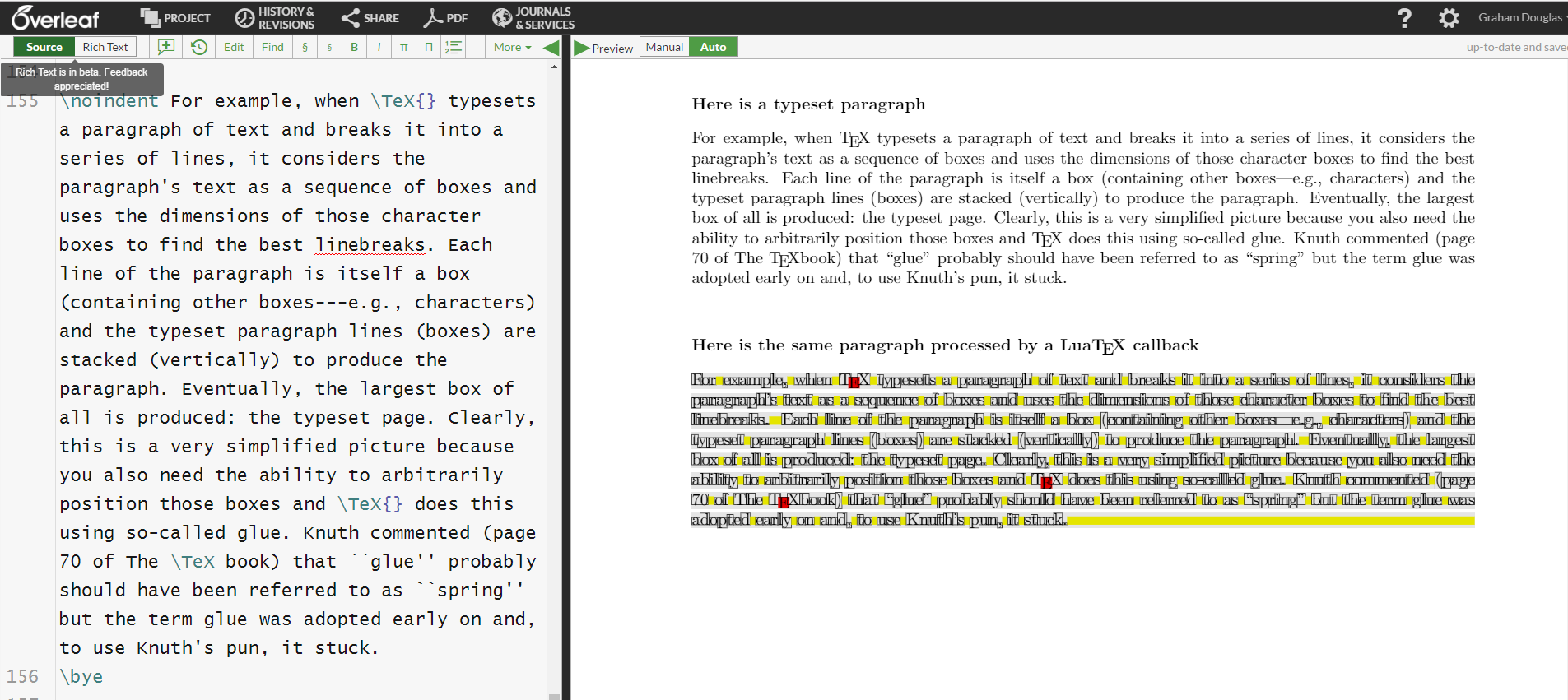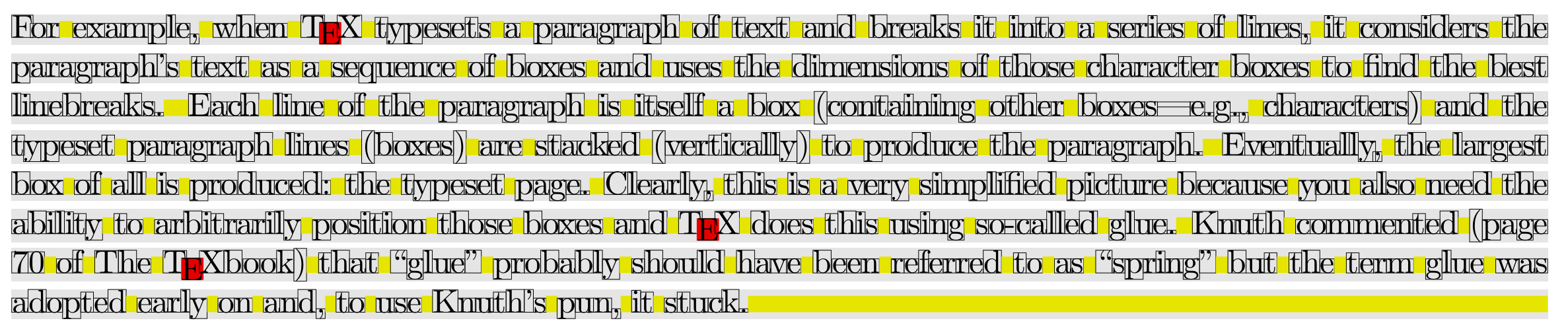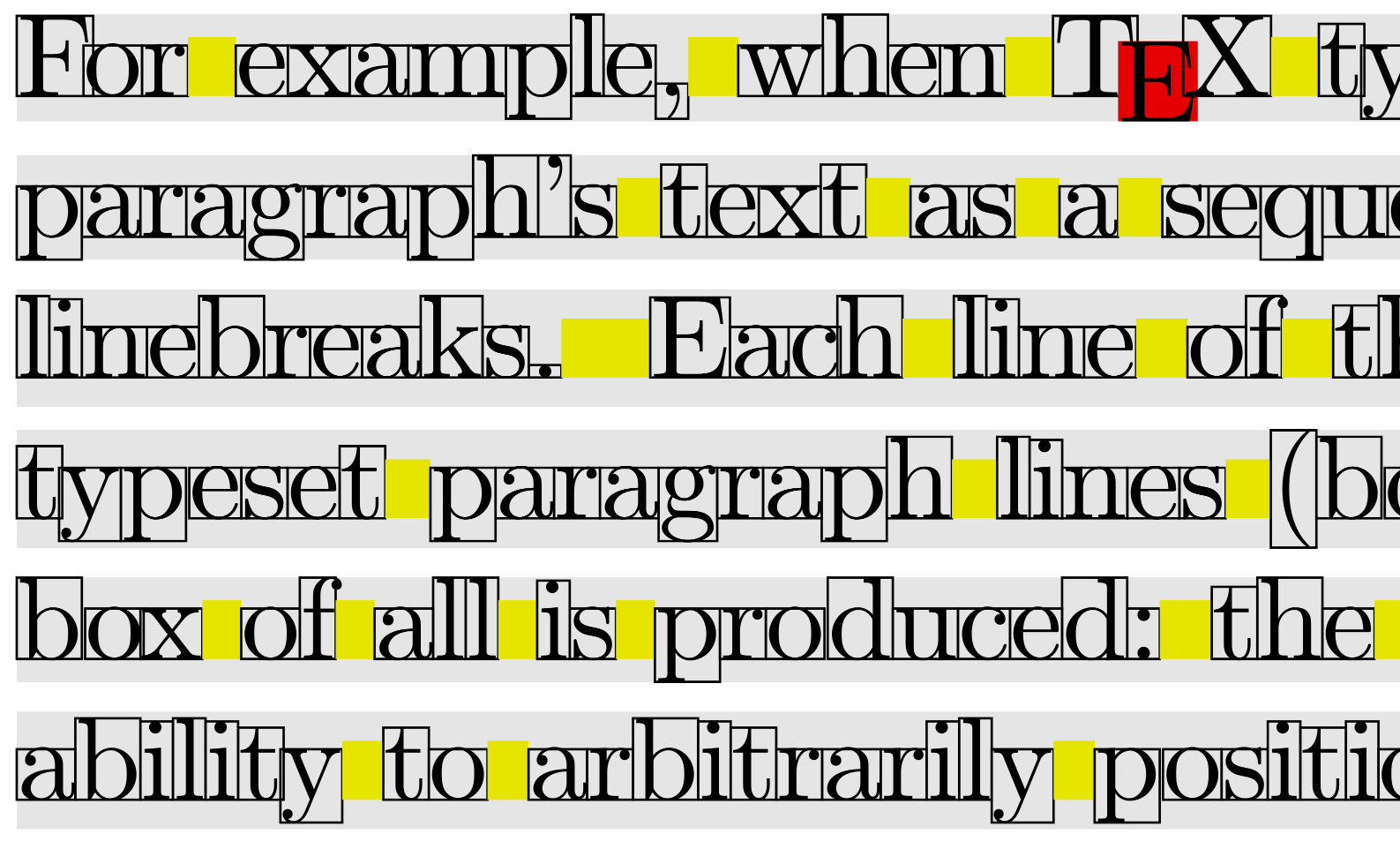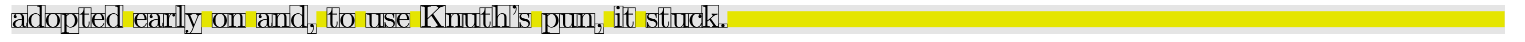Showing first {{hits.length}} results of {{hits_total}} for {{searchQueryText}}{{hits.length}} results for {{searchQueryText}}

No Search Results

Contents 1 An introduction for future articles 2 Back to the basics... 3 Visualizing a typeset paragraph using $$\mathrm{Lua}\mathrm\TeX$$ 3.1 Until the next post An introduction for future articles This post is a brief introduction to some key concepts/models involved in typesetting with $$\mathrm \TeX$$: boxes and glue—“setting the scene” for future articles that will go into much more detail. A forthcoming post will explore the low-level behaviour of $$\mathrm \TeX$$ boxes using $$\mathrm{Lua}\mathrm\TeX$$ to produce diagrams (node graphs) such as the following example:This node graph is produced from an \hbox{...} created with the following $$\mathrm \TeX$$ code:   \hbox to100pt{A\hskip4pt plus3pt minus 2pt B% \hskip 0pt plus 2fil C\hskip 0pt plus 2fill D\hskip 0pt plus 3fill} which looks like this (drawn with a bounding rectangle):These node graphs show the deep inner structure of $$\mathrm \TeX$$ boxes—reflecting how they are stored inside of $$\mathrm \TeX$$ and offer an invaluable graphical description that helps to better understand the behaviour of box-construction commands such as \hbox{...} or \vbox{...}. Back to the basics... Even as a new, or casual, $$\mathrm \LaTeX$$ user it doesn’t take long before you encounter the concept of $$\mathrm \TeX$$ “boxes” or that $$\mathrm \TeX$$ uses a “boxes and glue” model for its core typesetting activities. The typesetting algorithms of $$\mathrm \TeX$$ spend much of their time creating and then stacking horizontal and vertical lists of boxes. For example, when $$\mathrm \TeX$$ typesets a paragraph of text and breaks it into a series of lines, it considers the paragraph’s text as a stream or sequence of boxes and uses the width, height and depth of those character boxes (actually, glyphs) to find the best linebreaks and then add vertical space between the lines of text. Each typeset line of the paragraph is itself a box (containing other boxes—e.g., characters) and the typeset paragraph lines (boxes) are stacked vertically to produce the paragraph. Eventually, the largest box of all is produced: the typeset page. Clearly, this is an extremely simplified picture because you also need the ability to move and position those boxes and $$\mathrm \TeX$$ does this using so-called glue: a form of “flexible spacing”. Knuth has commented (page 70 of The $$\mathrm \TeX \mathrm{book}$$) that “glue” probably should have been referred to as “spring” but the term glue was adopted early on and, to use Knuth’s pun, it stuck. Visualizing a typeset paragraph using $$\mathrm{Lua}\mathrm\TeX$$ Using $$\mathrm{Lua}\mathrm\TeX$$, a typeset paragraph can be processed to display the components used to typeset it: the character boxes and the flexible glue used to put space between the words. We have created a simple $$\mathrm{Lua}\mathrm\TeX$$ (plain $$\mathrm \TeX$$) project to demonstrate this:The project’s code was written to illustrate this post and is not a full implementation of a paragraph “parser”—it ignores a number of $$\mathrm{Lua}\mathrm \TeX$$ node types and is designed purely to illustrate the ideas discussed in this post.In the zoomed image section, below, you can see the individual paragraph lines shaded as grey strips—note there is white space between the lines: vertical glue which $$\mathrm \TeX$$ inserts as it stacks them to form the paragraph. If you want to know more about the vertical glue inserted between lines in a typeset paragraph, use Google to find articles which discuss \baselineskip and \lineskiplimit.When $$\mathrm \TeX$$ reads and typesets a paragraph it converts interword space characters into blobs of glue whose precise value is a property of the font being used. During typesetting, these interword glues are stretched or shrunk by an amount considered necessary to achieve a pleasing linebreak. In the image above you should note the following:grey strips are the boundaries of the individual lines of the typeset paragraph;each character is shown within a box that defines its dimensions (as $$\mathrm \TeX$$ sees it);the yellow boxes show the glue that $$\mathrm \TeX$$ has inserted between the words in order to achieve a pleasing linebreak. Observe the following:the width of interword glue varies from line-to-line as $$\mathrm \TeX$$ stretches or shrinks it to achieve each linebreak;on the third line, the glue after the period character is wider than other glues on the same line.the red box shows where an explicit \hbox{...} was used. The definition of the \TeX command puts the E into an \hbox{...} so that it can be lowered to create the $$\mathrm \TeX$$ logo. At the end of our example paragraph there is a long strip of glue which is called \parfillskip: this is inserted by $$\mathrm \TeX$$ engines to fill up space on the last line.Until the next post We hope this post has provided a useful introduction to the principle of boxes and glue used within $$\mathrm \TeX$$. In a forthcoming post we’ll use $$\mathrm{Lua}\mathrm\TeX$$ to dig much deeper into the content of $$\mathrm \TeX$$ boxes—using node graphs such as the example shown at the start of this article.